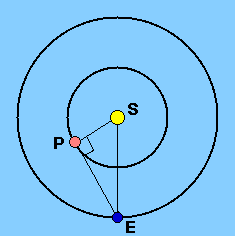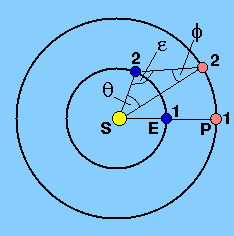Copernicus used his model of the solar system to obtain the distances to the inferior planets in units of the Earth's distance from the Sun (also known as an astronomical unit or AU), as shown in the left-hand panel of Figure 30. By carefully measuring the elongation (angle SEP, i.e. the angular distance between the Sun and the planet in the sky) on a series of nights around greatest elongation, it is possible to obtain a value for the greatest elongation, SEPmax. At this time, the angle between the line joining the planet to the Sun and the line joining the planet to the Earth is 90°. Hence

SP / SE = sin (SEPmax),

where the value of angle SEPmax is known. The quantity SP / SE is therefore the distance of the planet from the Sun in units of the Earth's distance from the Sun, i.e. in AU. There is an example problem which shows how Copernicus deduced the distances to Mercury and Venus in this way.

 figure 30: Measurement of the distance of an inferior planet (left-hand panel) and a superior planet (right-hand panel).If the planet is a superior one, the situation is slightly more complicated. Kepler was the first to realise it could be solved if the planet's synodic period, S, is known. If the planet's sidereal period is P days and the Earth's sidereal period is E days, then the planet moves at a rate of 360°/P degrees per day in its orbit and the Earth moves at a rate of 360°/E, as viewed from the Sun.

Let the planet be in opposition at position 1, as shown in the right-hand panel of Figure 30. After t days have elapsed, the planet will have moved through an angle 360°t/P, as viewed from the Sun, to position 2. In this time, the Earth will have moved through an angle 360°t/E from position 1 to position 2. The angle between the planet and the Earth at position 2, as viewed from the Sun,, is hence given by= (360°t/E) - (360°t/P) = 360°t(1/E - 1/P).

Recalling that, for a superior planet,

1/S = 1/E - 1/P

we obtain= 360°t/S.

Since t and S are both known,can be evaluated.

The elongation of the planet at position 1 is 180°, i.e. it is in opposition. At position 2, the elongation of the planet is reduced to- this value can be measured in the sky. Hence the anglecan be found from the relation= 180° --.

Using the sine formula of plane trigonometry, we have

sin/ SP = sin/ SE

or

SP / SE = sin/ sin,

giving the distance of the planet from Sun in units of the Earth's distance from the Sun. Proceeding in this way, it is thus possible to construct an accurate model of the solar system in terms of astronomical units without knowing the scale absolutely, as shown in the example problems. Such a model was first obtained by Kepler. It was a far more difficult problem, only satisfactorily solved with the advent of radar this century, to measure the scale (i.e. the distances of the planets in kilometres). The radar method of distance determination involves timing the interval between transmission of a radar pulse by a powerful radio telescope on Earth and the reception of its echo from the planet. Thus if EP, c and t are the Earth-planet distance, the velocity of radio waves and the time interval, respectively,

EP = ½ct.

Various corrections have to be made to derive the true distance. For example, the distance actually measured is the distance from the radio telescope to the surface of the planet, and not the distance between the Earth's centre and the planet's centre. The effect of the change in the speed of the radar pulse when passing through the Earth's atmosphere also has to be taken into account. With a knowledge of the distance in kilometres from the Earth of just one planet in the solar system (e.g. Venus), it is possible to determine the distance to all of the rest (and the size of the astronomical unit) in kilometres.